# Division of DecimalsPage 1

#### WATCH ALL SLIDES

Slide 1Dividing Decimals

Slide 2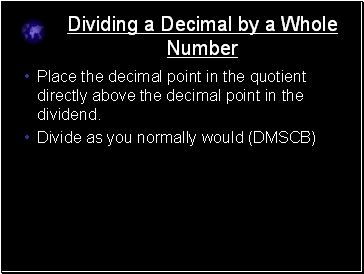## Dividing a Decimal by a Whole Number

Place the decimal point in the quotient directly above the decimal point in the dividend.

Divide as you normally would (DMSCB)

Slide 3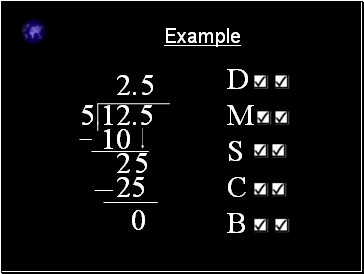Example

5

12.5

.

DMSCB

2

10

2

5

5

25

0

Slide 4Example 2

9

101.52

.

1

9

1

1

1

9

2

5

2

18

7

2

8

72

0

Slide 5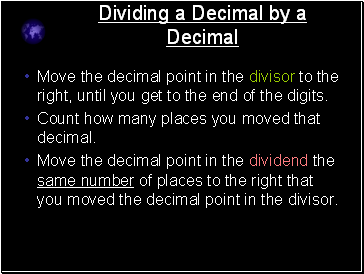## Dividing a Decimal by a Decimal

Move the decimal point in the divisor to the right, until you get to the end of the digits.

Count how many places you moved that decimal.

Move the decimal point in the dividend the same number of places to the right that you moved the decimal point in the divisor.

Slide 6Example

0.3

6.72

one space

03

67.2

New problem

Slide 7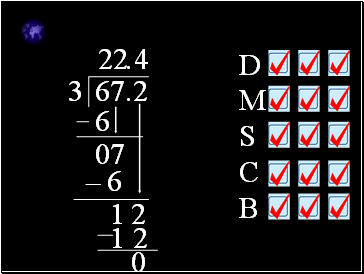3

67.2

DMSCB

.

2

6

0

7

2

6

1

2

4

1 2

0

Slide 8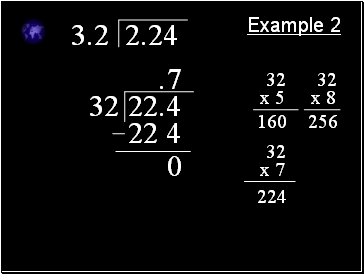Example 2

3.2

2.24

32

22.4

.

32

x 5

160

32

x 8

256

32

x 7

224

7

22 4

0

Slide 9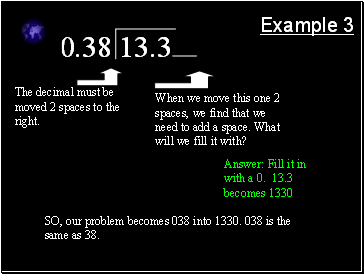Example 3

0.38

13.3

The decimal must be moved 2 spaces to the right.

When we move this one 2 spaces, we find that we need to add a space. What will we fill it with?

Answer: Fill it in with a 0. 13.3 becomes 1330

SO, our problem becomes 038 into 1330. 038 is the same as 38.

Slide 10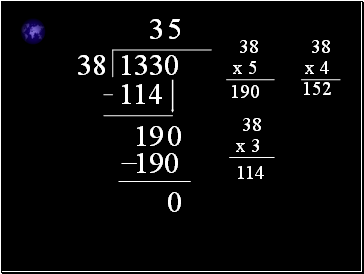38

1330

38

x 5

190

38

x 4

152

38

x 3

114

3

114

19

0

5

190

0

Slide 11Example 4

2.48

1.3392

248

133.92

Slide 12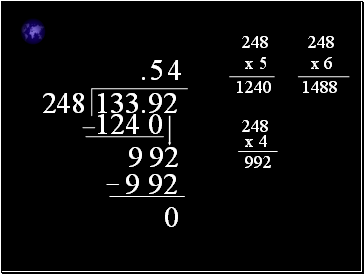248

133.92

.

248

x 5

1240

248

x 6

1488

5

124 0

9

9

2

248

x 4

992

4

9 92

0

Slide 13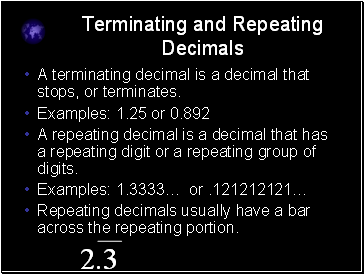Go to page:
1  2